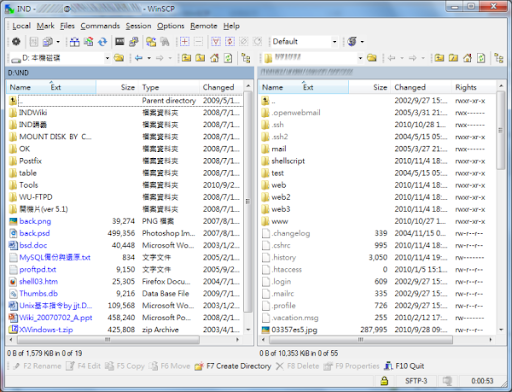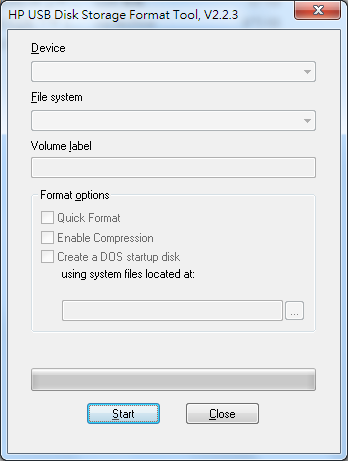# WinSCP – 在Windows上使用SCP傳輸檔案# Outlook Express 收件匣爆掉的解決方法

1. 進入OE，在左方的本機資料夾(或是看使用者有無其他的)新建立一個空匣，假如先取名為Backup。
2. 關閉OE，到使用者的郵件所在目錄，把剛才建立的 Backup.dbx 手動刪除。
3. 將 收件匣.dbx 改名為 Backup.dbx。
4. 此時再打開OE，因為收件匣不存在的話OE一定會建立一個新的，而這時去看Backup就可以看到剛才收件匣的信都在Backup裡。

# 利用 HP USB Disk Storage Format Tool 製作可開機的USB隨身碟``````function Skada:FormatNumber(number)
if number then
if self.db.profile.numberformat == 1 then
if number > 100000000 then
return  ("%02.2f億"):format(number / 100000000)
elseif number > 1000000 then
return  ("%d萬"):format(number / 10000)
elseif number > 10000 then
return  ("%02.1f萬"):format(number / 10000)
else
return number
end
else
return number
end
end
end``````

``````function Skada:FormatNumber(number)
if number then
if self.db.profile.numberformat == 1 then
if number > 100000000 then
return  ("%02.2f億"):format(number / 100000000)
elseif number > 1000000 then
return  ("%d萬"):format(number / 10000)
elseif number > 10000 then
return  ("%02.1f萬"):format(number / 10000)
else
return number
end
else
return number
end
end
end``````

# 好用的 xargs 指令

[cc no_cc=”1″]find . -name ‘config_db.php’ | xargs sed -i ‘s/\”root\”/\”loki\”/g'[/cc]